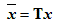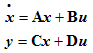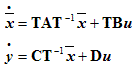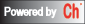Web-Based Control System Analysis and Design

## Coordinate transformation

#### DESCRIPTION:

The function performs a state coordinate transformationAssume the system has the following state equations,This function will make a similarity transformation on the state vector x, and returns the following model with equations,Please select a model to represent the system:

#### System Model:

State-space equations

#### System Type:

Continuous-time.
Discrete-time with the sampling time of   second